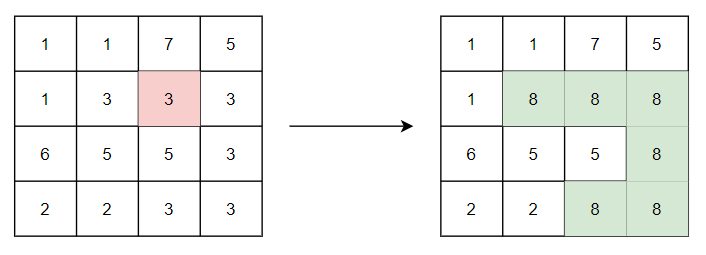# Flood Fill Algorithm

Posted: 12 Jan, 2021
Difficulty: Moderate

## PROBLEM STATEMENT

#### The image is represented in the form of a 2D array of size M * N. Each pixel in the image is a positive integer. Ninja has given you the coordinates (row and column) of a certain pixel and the new color value. You need to replace the color of the given pixel and all adjacent same-colored pixels with the new color.

##### Note:
``````Two pixels are adjacent if they are connected to each other in any of the four directions: up, down, left, or right.

Diagonal pixels are not considered adjacent.
``````
##### Example:
``````Consider the image of size 4*4, shown below (left). Let the coordinates of the starting pixel are (1, 2) and the new color is 8. The starting pixel, highlighted with red color, has a pixel value of 3.

On replacing the given pixel and all adjacent same-colored pixels with the new color we get the new image, shown below (right). The modified pixels are highlighted with green color.
``````##### Input Format:
``````The first line of input contains an integer ‘T’ representing the number of test cases.

The first line of every test case contains two space-separated integers ‘M’ and ‘N’ representing the number of rows and columns in the image.

Each of the next ‘M’ lines contains ‘N’ space-separated integers denoting the pixel values of the image.

The next line contains three space-separated integers ‘X’, ‘Y’, and ‘C’ denoting the row and column of the starting pixel and the new color, respectively.
``````
##### Output Format:
``````For each test case, the newly colored image is printed in the form of an M * N Matrix.
``````
##### Note:
``````You do not need to print anything, it has already been taken care of. Just implement the given function.
``````
##### Constraints:
``````1 <= T <= 100
1 <= M, N <= 50
0 <= X < M
0 <= Y < N
1 <= Image[i][j], C <= 10^5

Time Limit: 1 sec
``````Approach 1
• Starting from the given pixel let's traverse the image using recursion.
• The idea is to replace the colour of the starting pixel with the new colour and repeat the same procedure for the adjacent same coloured pixels.
• During the traversal, we do not replace the colour of the current pixel if:
• It has a different colour than the starting pixel.
• Or if it has already been replaced with a new colour.
• In this way, we generate a new image.
• This approach is the same as applying DFS on the given image.

Algorithm: